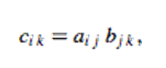Multiplication of matrices by C++ Programme:

THEORY:
The number of columns of the 1st matrix must equal to the number of rows of the 2nd matrix & the result will have the same number of rows as the 1st matrix, and the same number of columns as the 2nd matrix.

The product of two matrices A & B is defined asCODING:
#include<iostream.h>
#include<conio.h>
#include<math.h>
void main()
{
int a,b,c;
int i,j,k,sum;
clrscr();
cout<<"Enter the elements of 1st matrix:\n";
for(i=0;i<=2;i++)
{
for(j=0;j<=2;j++)
{
cin>>a[i][j];
}
}
cout<<"Enter the elements of 2nd matrix:\n";
for(i=0;i<=2;i++)
{
for(j=0;j<=2;j++)
{
cin>>b[i][j];
}
}
cout<<"The 1st matrix is :\n";
for(i=0;i<=2;i++)
{
for(j=0;j<=2;j++)
{
cout<<a[i][j]<<"\t";
}
cout<<endl;
}
cout<<"The 2nd matrix is :\n";
for(i=0;i<=2;i++)
{
for(j=0;j<=2;j++)
{
cout<<b[i][j]<<"\t";
}
cout<<endl;
}
for (i=0;i<=2;i++)
{
for(j=0;j<=2;j++)
{
sum=0;
for(k=0;k<=2;k++)
{
sum=sum+a[i][k]*b[k][j];
c[i][j]=sum;}
}
}
cout<<"The product of the two matrices is:\n";
for(i=0;i<=2;i++)
{
for(j=0;j<=2;j++)
{
cout<<c[i][j]<<"\t";
}
cout<<endl;
}
cout<<"\n Press any key to exit:";
getch();
}
OUTPUT: